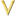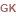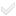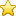# Fractal (Civ5 Type)

This page is a part of the Lua and UI Reference (Civ5).

# Static Methods

Methods are functions that belong to an object. Static methods are invoked through a dot, as in `Fractal.SomeMethod(<args>)`. When a dot is used the caller object is not implicitly provided as the first argument.`___________________________``Fractal` `Create(int width, int height, int grain, int fractalFlags, float xExponent, float yExponent)``Fractal` `CreateRifts(int regionWidth, int regionHeight, int continent_grain, table fracFlags, Fractal riftsFrac, float regionFracXExp, float regionFracYExp)`

# Instance Methods

Methods are functions that belong to an object. Instance methods are invoked through a colon, as in `caller:SomeMethod(<args>)`, where caller is an instance of Fractal.
A colon implictly passes the caller object as the first argument. That is, the former call is equivalent to this one: `caller.SomeMethod(caller, <args>)``___________________________``BuildRidges(int numPlates, table flags, int xExponent, int yExponent)``int` `GetHeight(int x, int y = nil)`

# Used by

 `Fractal` `Fractal.Create(int width, int height, int grain, int fractalFlags, float xExponent, float yExponent)` `Fractal` `Fractal.CreateRifts(int regionWidth, int regionHeight, int continent_grain, table fracFlags, Fractal riftsFrac, float regionFracXExp, float regionFracYExp)`

The initial version of this page was created by the Civ5 API Bot, see the Civ5 API Reference FAQ. Some of the texts come from the 2kgames' wiki and most of code samples are copyrighted to Firaxis.
Functions' signatures were either copied from the 2kgames' wiki, or infered from the Lua source files and the binaries. Errors are possible.
Contributors may find help in the Contributors guide to the Civ5 API.